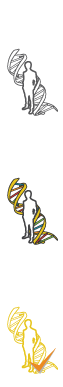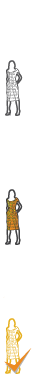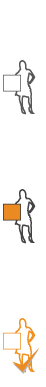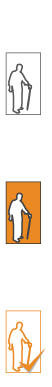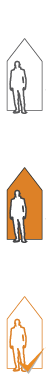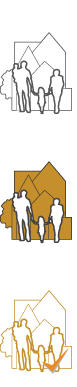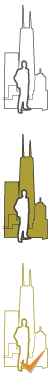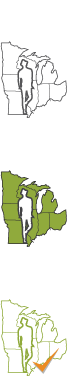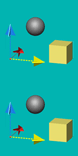3D Geometry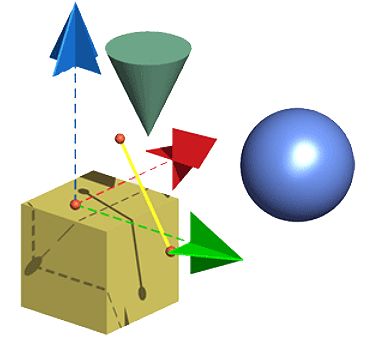Geometry is the mathematical study of shapes, figures and positions in space. Geo refers to the earth and ometry refers to measure. From ancient times, people used geometry to determine and mark locations on land and in the cosmos.

All geometry begins with the point. The point is the smallest unit of geometry. It takes three points to make a line: a beginning, a middle and an end. Lines form shapes and shapes exist in planes. Shapes are in planes and have length (x) and height (y).

A triangle has three sides, a cube is four-sided and a circle is all points in a plane at a fixed distance (radius) from a center point. Add the dimension width (z) to these shapes and you have a volume. Triangles become pyramids, squares become cubes and circles become spheres. Geometry locates positions in space (x, y, z) with points, lines, and angles. Make the invisible visible! Take measure of the earth!

Activity 1 – Skyline GeometryChoose one of the city skylines or photograph your own hometown. Find and outline at least one example of each two dimensional shapes and three dimensional volumes of the following:

1. triangle
2. rectangle
3. square
4. circle
5. regular polygon
6. irregular polygon
7. concave shape
8. pyramid
9. cube
10. sphere
11. point
12. line
13. plane

Activity 2 – Polygon and Volume Power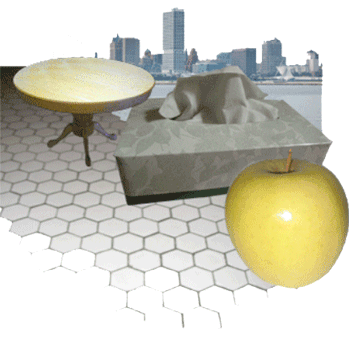Examine geometry around your home. Find examples of two dimensional geometry: triangles, rectangles and pentagons anywhere you can. Then, find examples of three dimensional geometry: spheres, pyramids, cylinders and cubes.

Look at the floor tile, the design of a door knob or even your soccer ball. Photograph or sketch what you find, then copy them, label them and paste the shapes in your journal. Think about how to fold a 3-dimensional shape from a flat piece of paper. Is it even possible? Start with a square shape and draw all six sides on a flat sheet of construction paper. Cut and fold your design into a cube. Then work with a triangle to make a pyramid. Finally, be brave! Try to draw and fold more complex shapes into regular solids.

Activity 3 – Volume Power In Your Neighborhood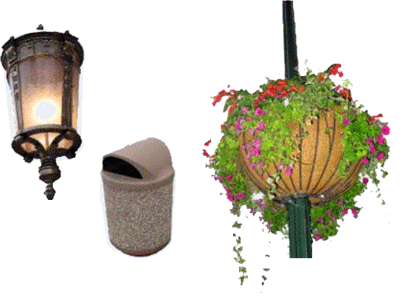Take activity 2 out into your neighborhood. This time, look for three-dimensional volumes. Find examples of pyramids, cubes, and spheres anywhere you can. Look closely; they are everywhere around you. A light pole or a mailbox, or look into the eave of your house; almost everything is a 3-dimensional shape. Sketch and photograph your discoveries. Next, paste them in your journal to make a map of your neighborhood. See if your friends can guess where you found each shape.

There is a language in shape!

Activity 4 – Digital 3D!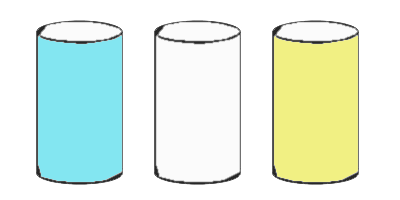Using GoogleSketchUp start drawing 3 dimensional geometry!

Plot a circle; pull up a cylinder!

Review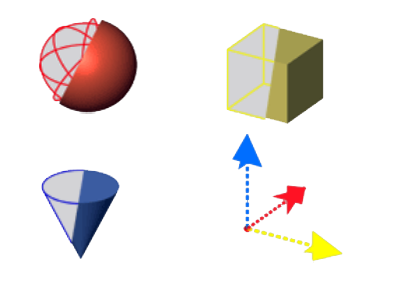• How are the dimensions of a cube described?
• How many sides can a pyramid have?
• What is the strongest shape?
• The Earth is a perfect sphere:
• Is a dome 3-dimensional or 2-dimensional?